Скачать презентацию ICDCS 2009 June 24 2009 Montreal Stochastic Multicast

216d2878c2e06fdcf527fb721d5eca6c.ppt

• Количество слайдов: 24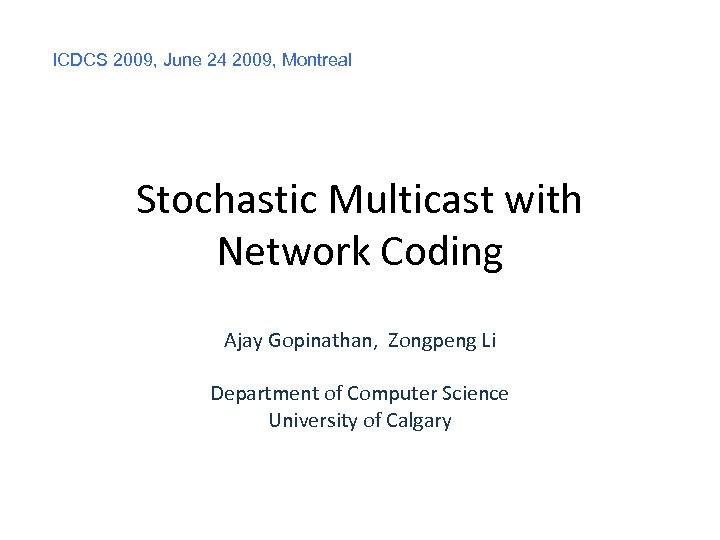ICDCS 2009, June 24 2009, Montreal Stochastic Multicast with Network Coding Ajay Gopinathan, Zongpeng Li Department of Computer Science University of Calgary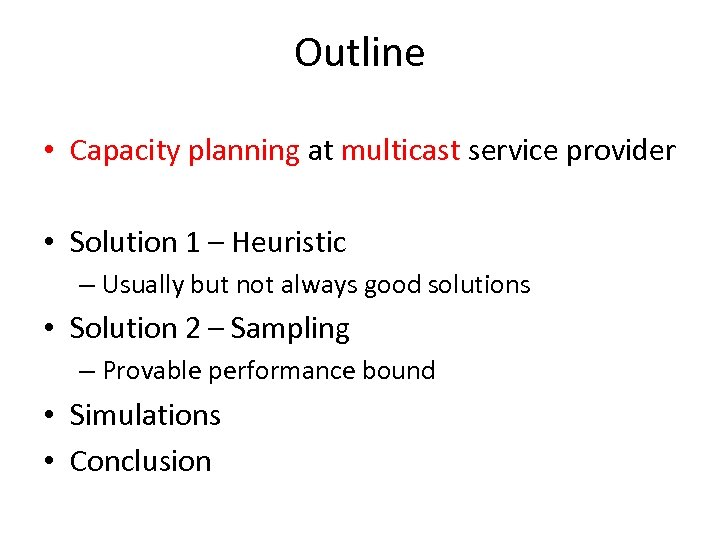Outline • Capacity planning at multicast service provider • Solution 1 – Heuristic – Usually but not always good solutions • Solution 2 – Sampling – Provable performance bound • Simulations • Conclusion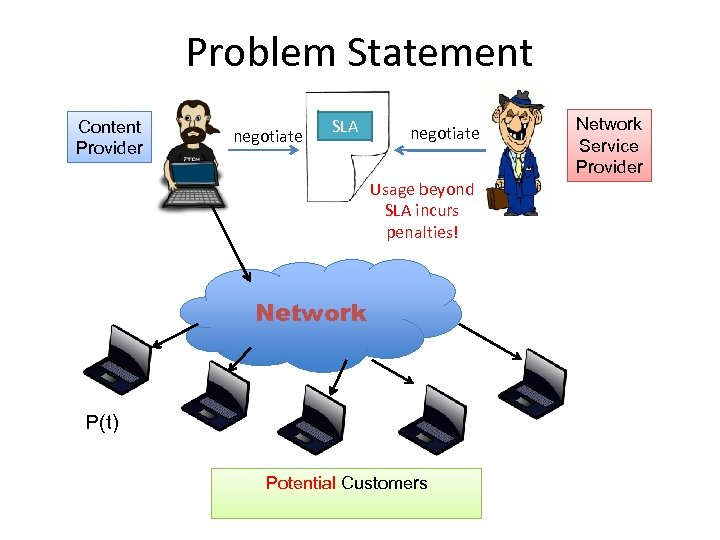Problem Statement Content Provider negotiate SLA negotiate Usage beyond SLA incurs penalties! Network P(t) Potential Customers Network Service Provider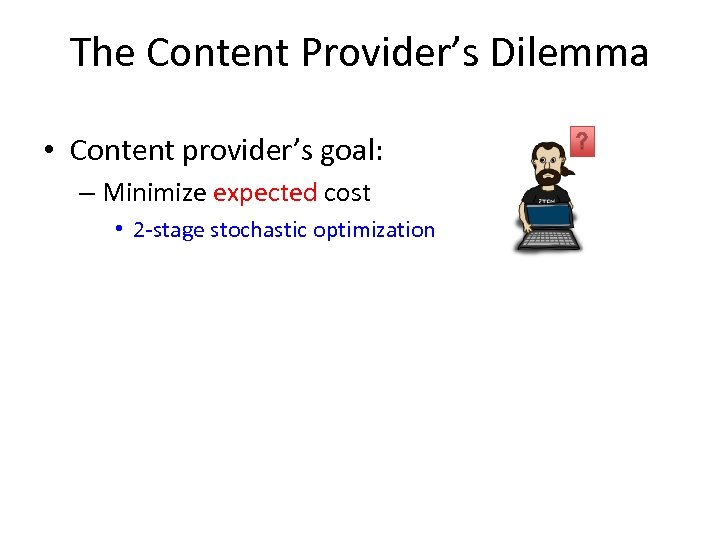The Content Provider’s Dilemma • Content provider’s goal: – Minimize expected cost • 2 -stage stochastic optimization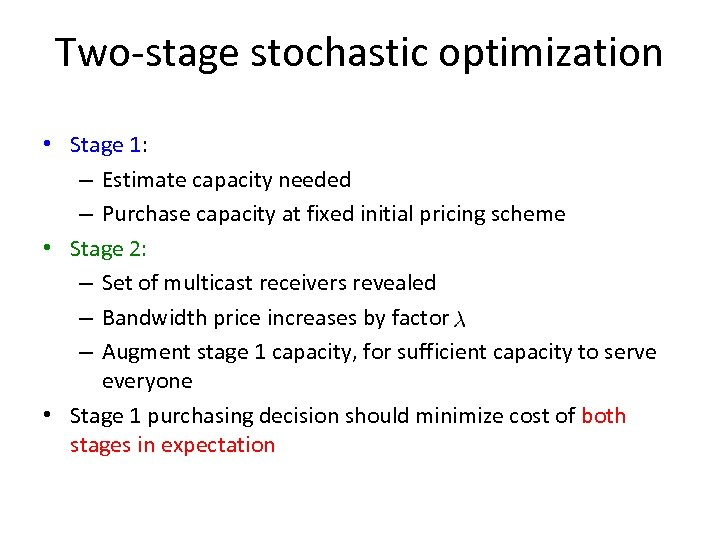Two-stage stochastic optimization • Stage 1: – Estimate capacity needed – Purchase capacity at fixed initial pricing scheme • Stage 2: – Set of multicast receivers revealed – Bandwidth price increases by factor – Augment stage 1 capacity, for sufficient capacity to serve everyone • Stage 1 purchasing decision should minimize cost of both stages in expectation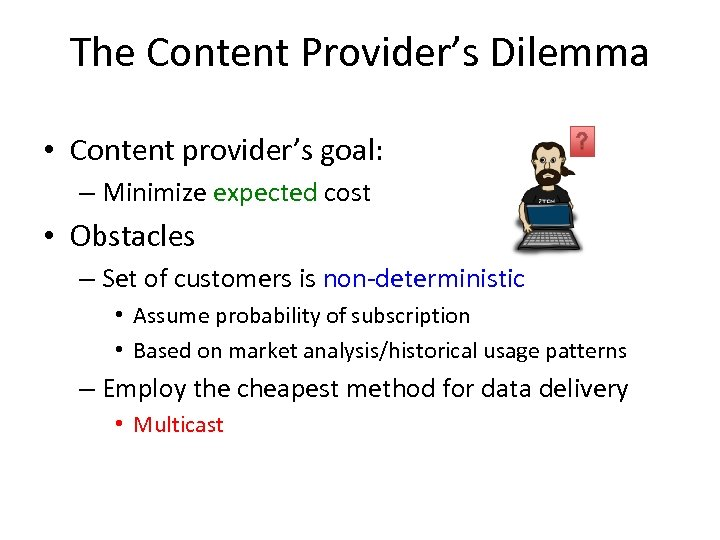The Content Provider’s Dilemma • Content provider’s goal: – Minimize expected cost • Obstacles – Set of customers is non-deterministic • Assume probability of subscription • Based on market analysis/historical usage patterns – Employ the cheapest method for data delivery • Multicast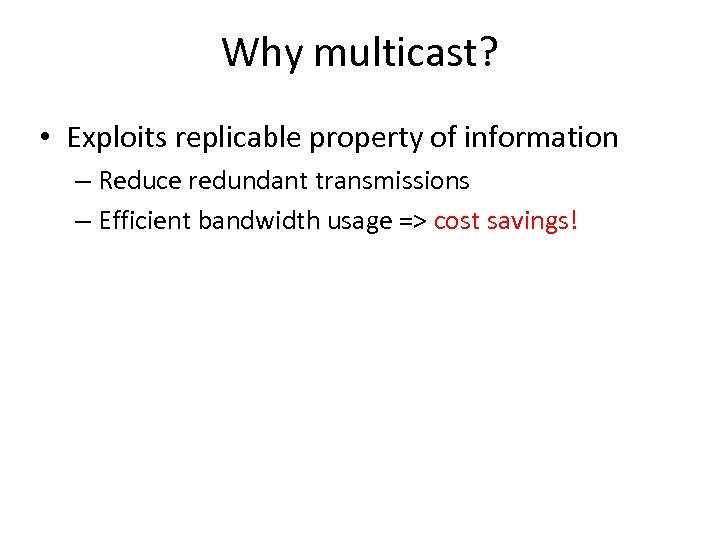Why multicast? • Exploits replicable property of information – Reduce redundant transmissions – Efficient bandwidth usage => cost savings!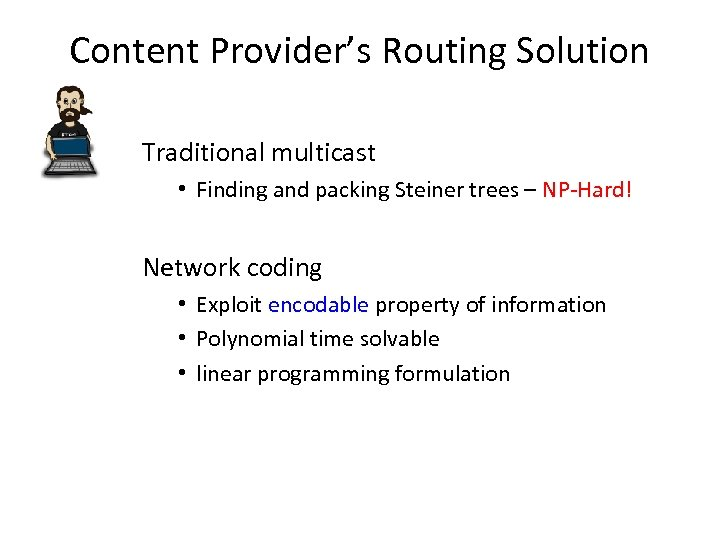Content Provider’s Routing Solution Traditional multicast • Finding and packing Steiner trees – NP-Hard! Network coding • Exploit encodable property of information • Polynomial time solvable • linear programming formulation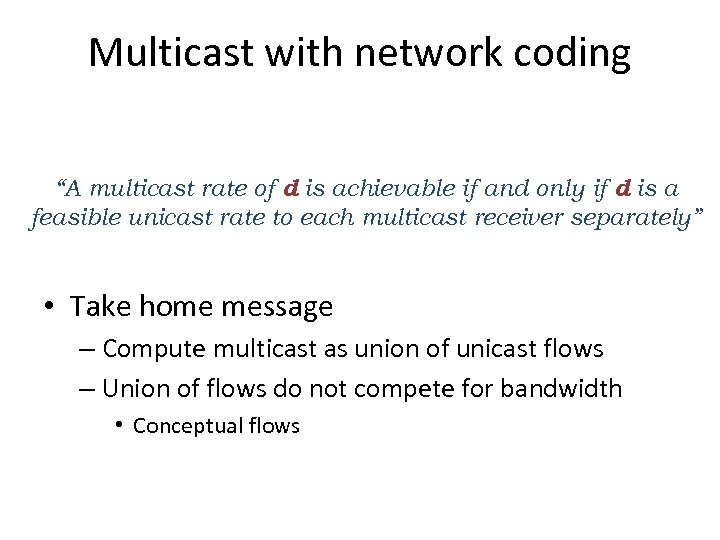Multicast with network coding “A multicast rate of d is achievable if and only if d is a feasible unicast rate to each multicast receiver separately” • Take home message – Compute multicast as union of unicast flows – Union of flows do not compete for bandwidth • Conceptual flows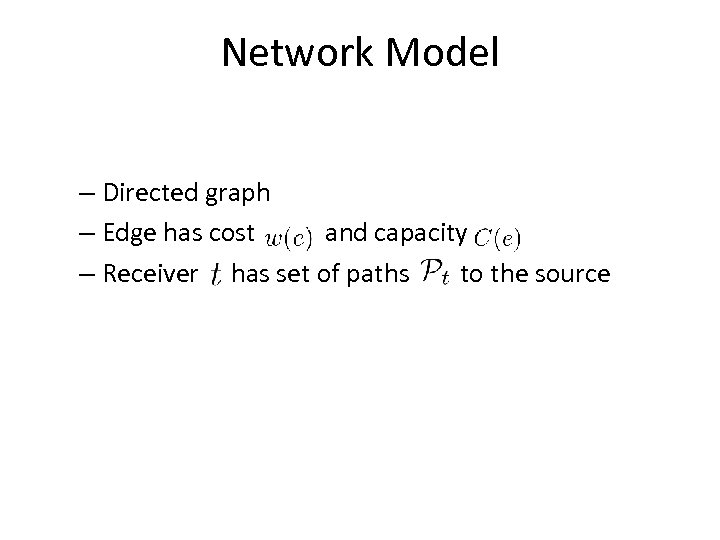Network Model – Directed graph – Edge has cost and capacity – Receiver has set of paths to the source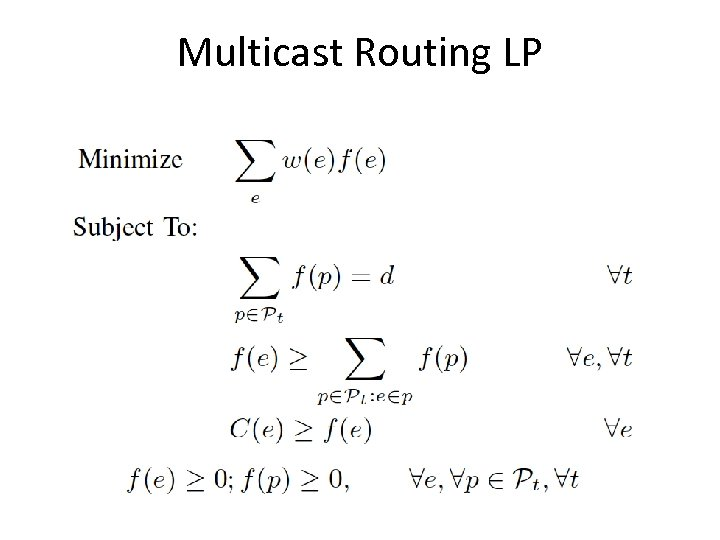Multicast Routing LP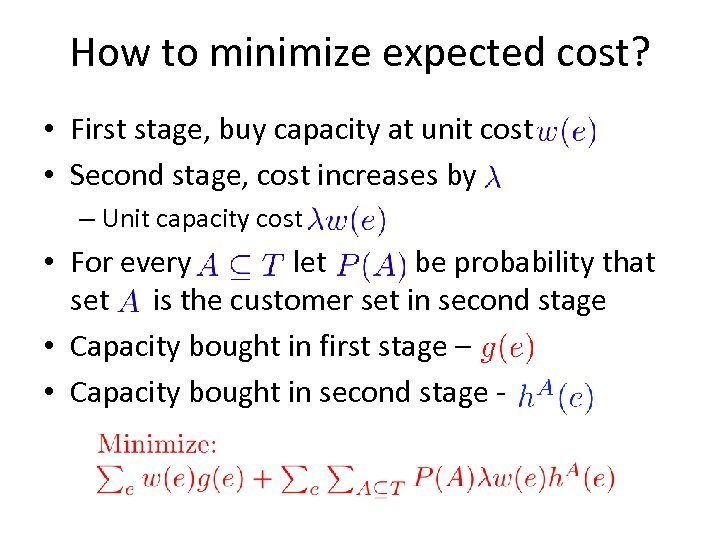How to minimize expected cost? • First stage, buy capacity at unit cost • Second stage, cost increases by – Unit capacity cost • For every let be probability that set is the customer set in second stage • Capacity bought in first stage – • Capacity bought in second stage -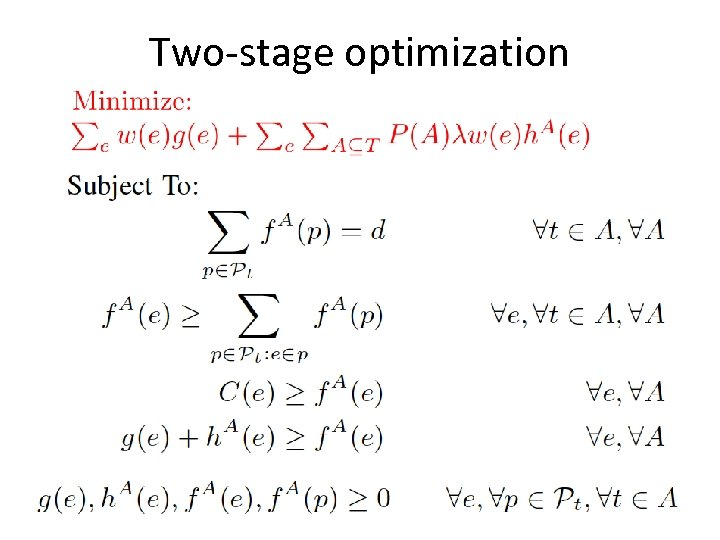Two-stage optimization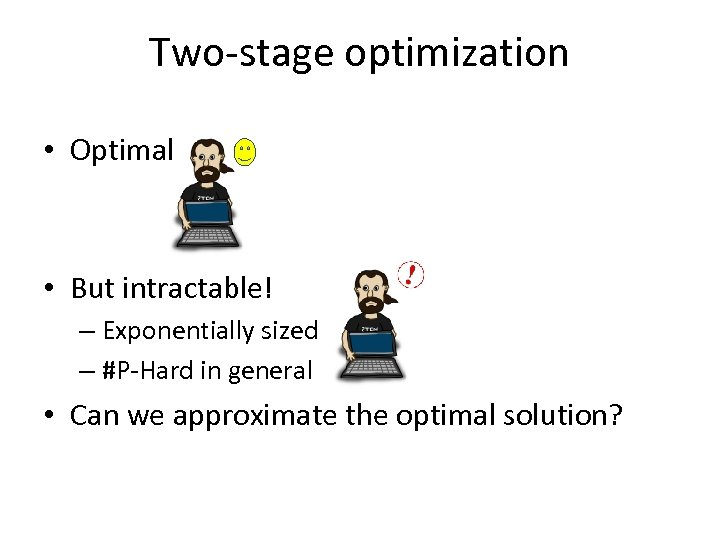Two-stage optimization • Optimal • But intractable! – Exponentially sized – #P-Hard in general • Can we approximate the optimal solution?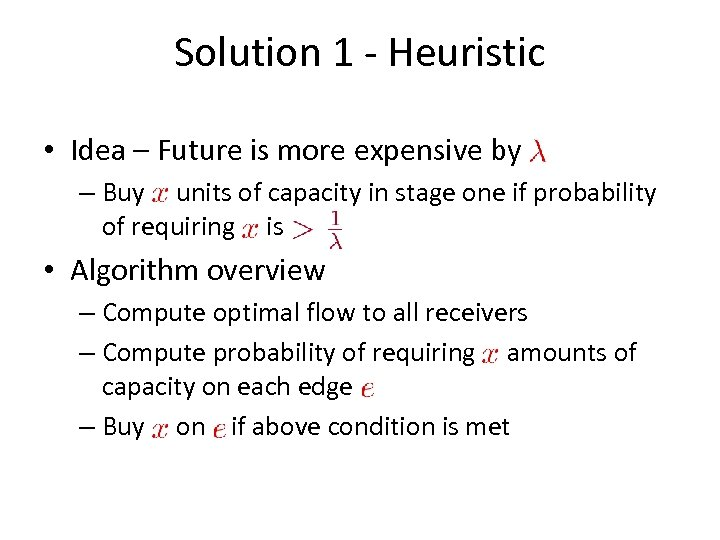Solution 1 - Heuristic • Idea – Future is more expensive by – Buy units of capacity in stage one if probability of requiring is • Algorithm overview – Compute optimal flow to all receivers – Compute probability of requiring amounts of capacity on each edge – Buy on if above condition is met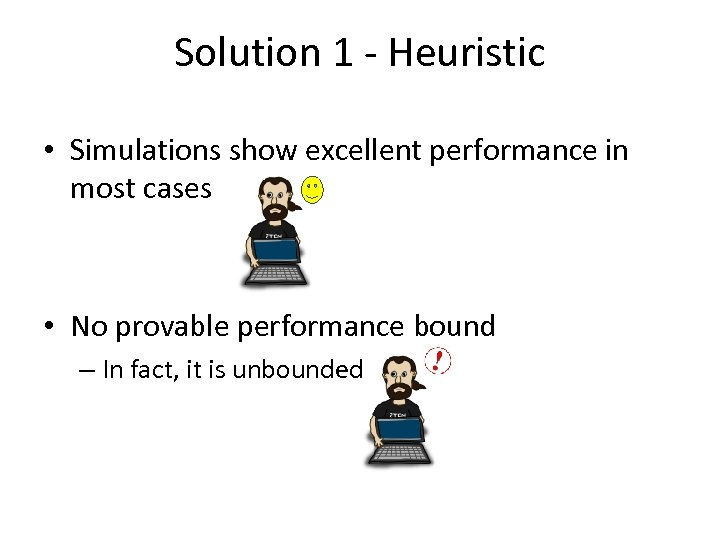Solution 1 - Heuristic • Simulations show excellent performance in most cases • No provable performance bound – In fact, it is unbounded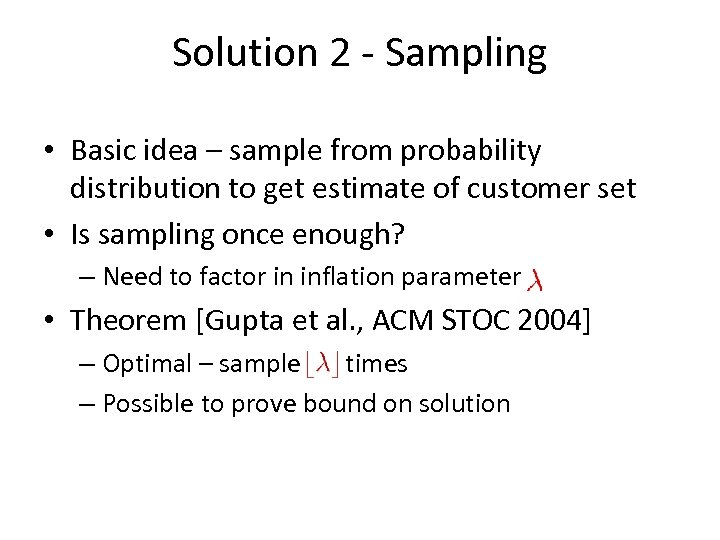Solution 2 - Sampling • Basic idea – sample from probability distribution to get estimate of customer set • Is sampling once enough? – Need to factor in inflation parameter • Theorem [Gupta et al. , ACM STOC 2004] – Optimal – sample times – Possible to prove bound on solution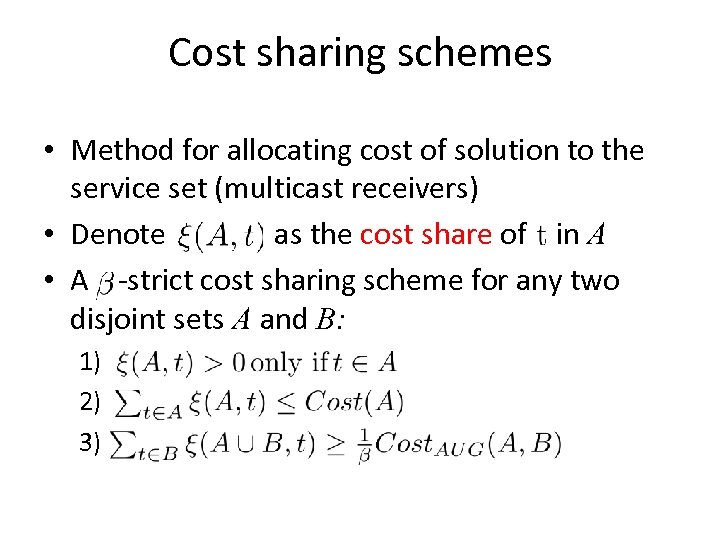Cost sharing schemes • Method for allocating cost of solution to the service set (multicast receivers) • Denote as the cost share of in A • A -strict cost sharing scheme for any two disjoint sets A and B: 1) 2) 3)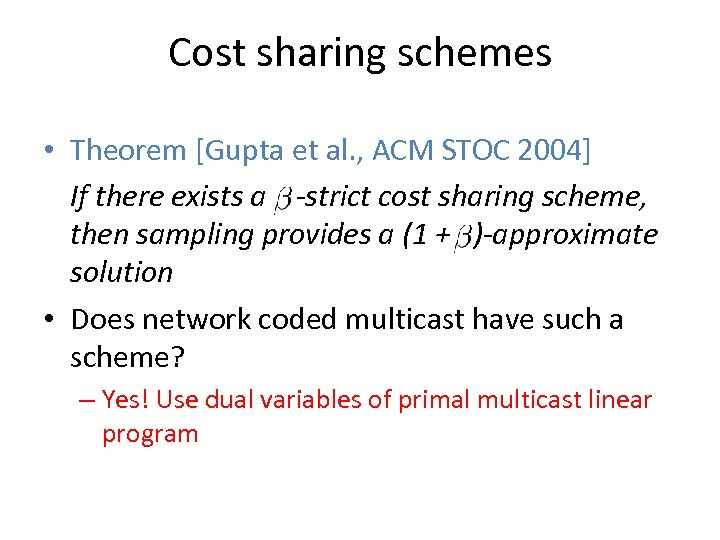Cost sharing schemes • Theorem [Gupta et al. , ACM STOC 2004] If there exists a -strict cost sharing scheme, then sampling provides a (1 + )-approximate solution • Does network coded multicast have such a scheme? – Yes! Use dual variables of primal multicast linear program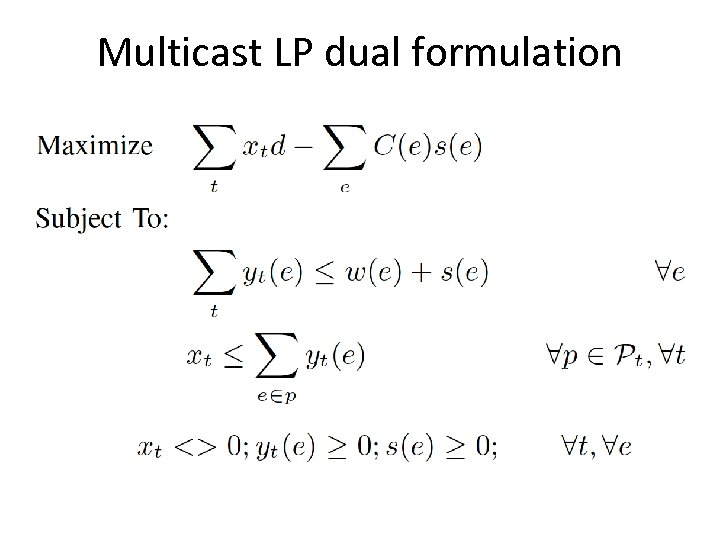Multicast LP dual formulation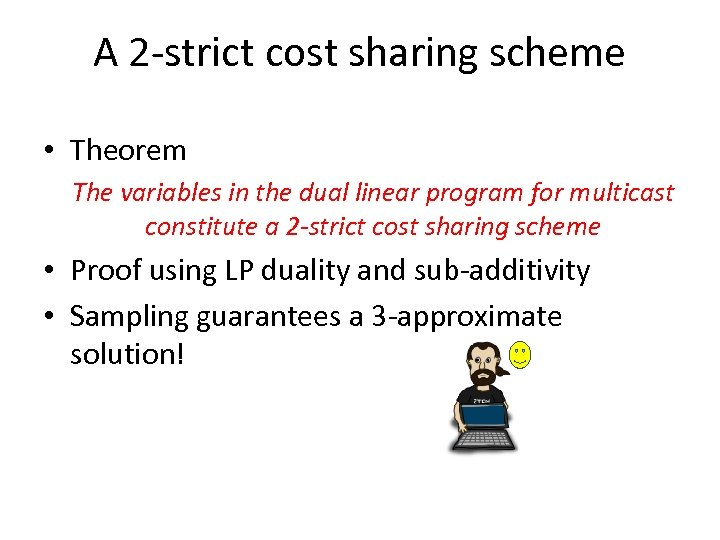A 2 -strict cost sharing scheme • Theorem The variables in the dual linear program for multicast constitute a 2 -strict cost sharing scheme • Proof using LP duality and sub-additivity • Sampling guarantees a 3 -approximate solution!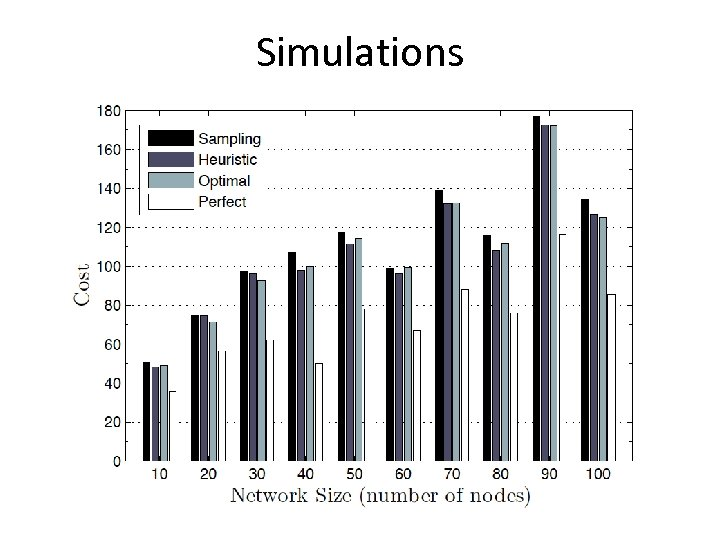Simulations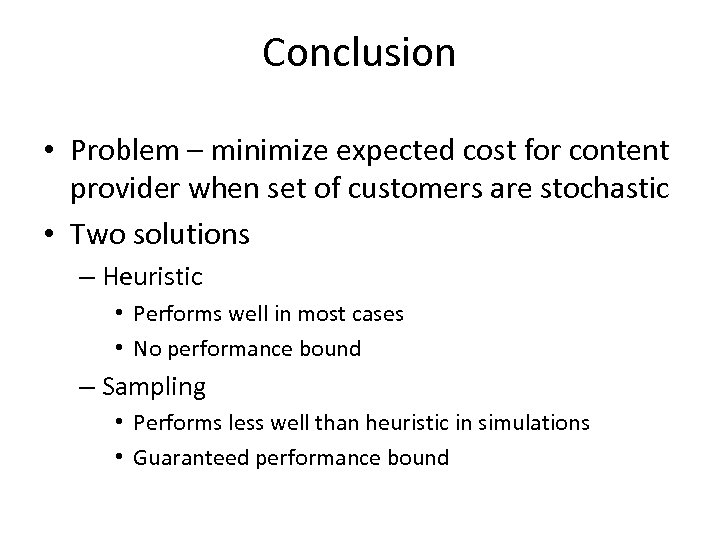Conclusion • Problem – minimize expected cost for content provider when set of customers are stochastic • Two solutions – Heuristic • Performs well in most cases • No performance bound – Sampling • Performs less well than heuristic in simulations • Guaranteed performance bound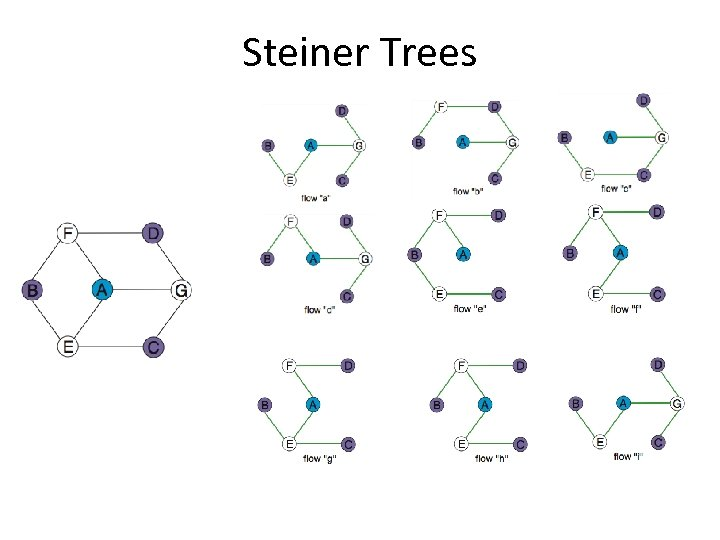Steiner Trees